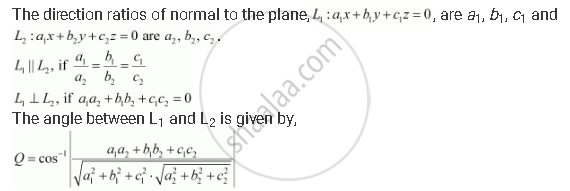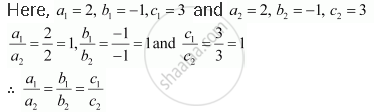Share

# In the Following Cases, Determine Whether the Given Planes Are Parallel Or Perpendicular, and in Case They Are Neither, Find the Angles Between Them. 2x – Y + 3z – 1 = 0 and 2x – Y + 3z + 3 = 0 - CBSE (Science) Class 12 - Mathematics

#### Question

In the following cases, determine whether the given planes are parallel or perpendicular, and in case they are neither, find the angles between them.

2x – y + 3z – 1 = 0 and 2x – y + 3z + 3 = 0

#### SolutionThe equations of the planes are  2x – y + 3z – 1 = 0 and 2x – y + 3z + 3 = 0Thus, the given lines are parallel to each other.

Is there an error in this question or solution?

#### APPEARS IN

NCERT Solution for Mathematics Textbook for Class 12 (2018 to Current)
Chapter 11: Three Dimensional Geometry
Q: 13.4 | Page no. 494

#### Video TutorialsVIEW ALL 

Solution In the Following Cases, Determine Whether the Given Planes Are Parallel Or Perpendicular, and in Case They Are Neither, Find the Angles Between Them. 2x – Y + 3z – 1 = 0 and 2x – Y + 3z + 3 = 0 Concept: Angle Between Two Planes.
S